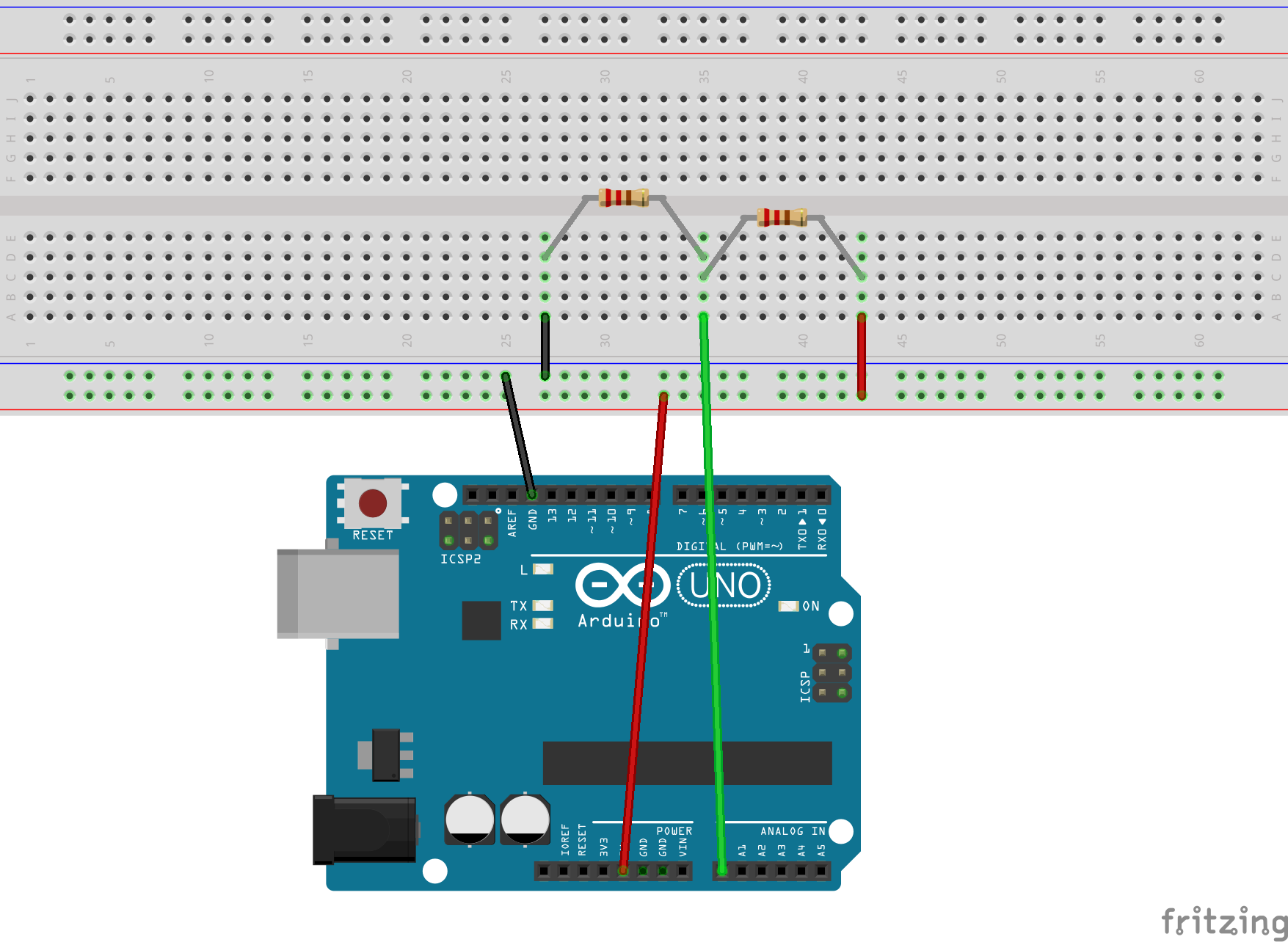# Developing in C for the ATmega328P: Function - analogRead()

Where I describe the function - analogRead().

## Introduction

The function analogRead() is similar to digitalRead(), in the sense, it takes a pin number as a parameter and returns the pin’s value. In the case of analogRead, the value will be a number between 0 and 1024. With the range of numbers corresponding to the range of voltages on the pin, typically between 0V and 5V.

## Digital vs Analog

In the microcontroller world, there are two methods of measurement, digital and analog. The former identifies a binary value, HIGH or LOW. The latter describes a linear range of values, based on the voltage which appears on the pin and the number of bits of the analog-to-digital controller (ADC) on the microcontroller. For example, the Uno has a 10-bit ADC, which means the value may range from 0-1024. One more element defining the value is the analog reference (AREF) voltage, which defines the highest voltage, the value 1024 will represent. Typically, (and in our case) this value will be 5V. (There are ways to define it as 1.1V or to an external voltage on a specific pin.) Therefore, the range of voltages which can be returned by analogRead() are 0V to 5V, and each step is 5V/1024 or .00488V or 4.88mV.

### Boring example

The image below illustrates a boring use of analogRead(). We’re using a simple voltage divider (two resistors across a voltage) to measure a voltage on pin A0. Why is this boring? The voltage won’t change, so there’s little value of measuring it more than once!Uno and a resistor voltage divider

Large Version to see detail

### Fun example

If we replace the two resistors with a floating wire, things become more interesting. A floating wire means while one end of the wire is connected to an Uno pin, the other end “floats” and isn’t connected to anything. This means it will have a random voltage based on what is going on around it. Try waving your hand around it, touch the wire or just leave it and watch the voltage change.Uno and a floating wire to show random voltages

Large Version to see detail

This code example will read pin A0 once per second. (This is a fun experiment where we plug a longish wire (~ 2") into the connector at pin A0 and watch the voltage.)

 `````` 1 2 3 4 5 6 7 8 9 10 11 12 13 14 15 16 17 18 19 20 21 22 23 24 `````` ``````// analogRead - Read an analog voltage on an analog pin (A0-A5) // Reference voltage is assumed to be 5V, each step 5V/1024 or .00488 #include #include "uart.h" #include "delay.h" #include "pinMode.h" #include "analogRead.h" int main (void) { init_serial(); const uint8_t analog_pin = A0; puts("Testing analogRead"); while(1) { uint16_t analog_value = analogRead(analog_pin); float voltage = (analog_value * .00488); printf("Pin: %u Value: %u Voltage: %f \n",\ analog_pin, analog_value, voltage); delay(1000); } return(0); }``````

### Practical example

If we replace the wire with a potentiometer, things become more practical. A potentiometer looks like two resistors above, however, you are able to turn a knob and the ratio between the two resistors changes. With typical potentiometers (or pots), the change ranges from 0-100%, so as we rotate the knob, the change in voltage ranges from the voltage on the left pin (0 %) to the voltage on the right pin(100%), and we are measuring using the pin in the middle.